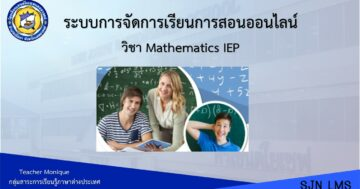# Mathematics IEP M.2 2nd Semester## เกี่ยวกับคอร์ส

Students are able to use the knowledge of geometry and tools such as a compass, straightedge, the Geometer’s Sketchpad or other dynamic geometric programs to construct geometric shapes; apply the knowledge of constructing to solve the problems in real life; use the knowledge about parallel lines and triangles to solve the mathematical problems; understand and use the properties of an equilateral triangle to solve the mathematical problems and the problems in real life; understand and use the knowledge in statistic to present the data and analyze the data from a dot plot, a stem and leaf plot, a histogram and mean; interpret the relationship including applying the statistic in real life by using an appropriate technology; understand and use the factorization of polynomials with 2 degree to solve mathematical problems.

### What Will You Learn?

• These are for the students to learn; to develop the skills in thinking process, calculation, problem solving, reasoning, and mathematical interpretation to creatively used in daily life; to appreciate and have good attitude towards mathematics; to be able to work systematically and thoroughly with responsibility, judgment, creativity and self-confidence.

00:00
00:00

00:00
00:00

### Student Ratings & ReviewsNo Review Yet